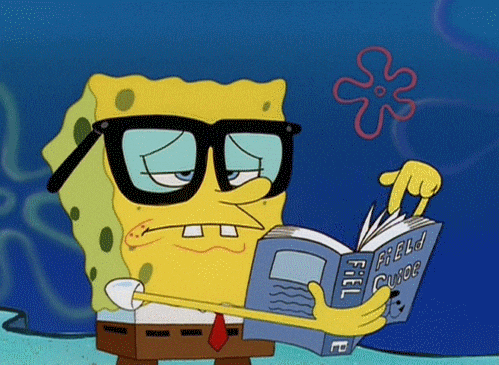# Using SDL2: Controlling our Stick Figure

###Noah11012 github logo Oct 31 '18・4 min read

Last time, our stick figure friend moved across the window from the left to the right. This time, we'll be taking control by using the keyboard to move the image wherever we want.

Before I made this post, I did some refactoring including making a new class for our image called `StickFigure`, placing the new class inside `Application` and replaced all instances of `NULL` with `nullptr`. It's been with the language since C++ 11 and basically, it's a pointer literal.

Here's all the files with the changes I made:

Application.hpp:

``````class Application
{
public:
Application();
~Application();

void loop();
void update(double delta_time);
void draw();
private:
StickFigure m_stick_figure;

SDL_Window  *m_window;
SDL_Surface *m_window_surface;
SDL_Event    m_window_event;
};
``````

Application.cpp:

``````void Application::update(double delta_time)
{
m_stick_figure.update(delta_time);
}

void Application::draw()
{
SDL_FillRect(m_window_surface, nullptr, SDL_MapRGB(m_window_surface->format, 0, 0, 0));

m_stick_figure.draw(m_window_surface);

SDL_UpdateWindowSurface(m_window);
}
``````

stick_figure.hpp:

``````#pragma once

#include <SDL2/SDL.h>

class StickFigure
{
public:
StickFigure();
~StickFigure() = default;

void update(double delta_time);
void draw(SDL_Surface *window_surface);
private:
SDL_Surface *m_image;
SDL_Rect     m_position;
double       m_x;
double       m_y;
};
``````

stick_figure.cpp:

``````#include "stick_figure.hpp"

StickFigure::StickFigure()
{

m_position.x = 0;
m_position.y = 0;
m_position.w = 22;
m_position.h = 43;

m_x = 0.0;
m_y = 0.0;
}

void StickFigure::update(double delta_time)
{
m_x = m_x + (5.0 * delta_time);
m_position.x = m_x;
}

void StickFigure::draw(SDL_Surface *window_surface)
{
SDL_BlitSurface(m_image, nullptr, window_surface, &m_position);
}
``````

One thing I want your attention drawn to is now that `update()` and `draw()` in `Application` don't update the position of the stick figure or draw it to the screen directly. That is `m_stick_figure`'s responsibility now. Instead, we call `update()` and `draw()` on a `StickFigure` object like `m_stick_figure`. This way, each class is now responsible for their own management.

## Keyboard input

We want to use the conventional WASD keys to move our stick figure. We need to know what buttons have been pressed for this to work. SDL2 gives us a function that returns an array of keys, sort of like a snapshot of a keyboard.

So, what is the function's name?

It's called `SDL_GetKeyboardState()` and it takes a pointer to an `int` and if non-null, gives the number of keys on your keyboard. We won't be using this, so we will pass in `nullptr`.

This function returns an array of constant `Uint8` or unsigned `char`s. Before we use this function, we'll add a function called `handle_events()` to `StickFigure` to, you guessed it, handle any inputs that the `Application` class may encounter.

``````void handle_events(SDL_Event const &event);
``````

In addition to our new method, I'll add an enumeration class to hold the different directions this image can go.

``````enum class Direction
{
NONE,
UP,
DOWN,
LEFT,
RIGHT
};

...

private:
Direction    m_direction;
``````

What is an enumeration class?

I won't be explaining it, but you can view this Stackoverflow Post.

We can index into this array that SDL returns to us by using an `SDL_Scancode` enumeration. If at one of these indices is a 1 then it's pressed, otherwise, 0.

In the `handle_update()` method, we check to see if a key is pressed and if so, which one is it. We then set the direction accordingly.

``````switch(event.type)
{
case SDL_KEYDOWN:
Uint8 const *keys = SDL_GetKeyboardState(nullptr);

if(keys[SDL_SCANCODE_W] == 1)
m_direction = Direction::UP;
else if(keys[SDL_SCANCODE_S] == 1)
m_direction = Direction::DOWN;
else if(keys[SDL_SCANCODE_A] == 1)
m_direction = Direction::LEFT;
else if(keys[SDL_SCANCODE_D] == 1)
m_direction = Direction::RIGHT;
break;
}
``````

In the `Application` class's `loop()` method, we pass in `m_window_event` to `StickFigure::hande_input()`

``````while(SDL_PollEvent(&m_window_event) > 0)
{
m_stick_figure.handle_events(m_window_event);
switch(m_window_event.type)
{
case SDL_QUIT:
keep_window_open = false;
break;
}
}
``````

The method `update()` in the class `StickFigure` moves the image depending what value `m_direction` is set to.

``````switch(m_direction)
{
case Direction::NONE:
m_x += 0.0;
m_y += 0.0;
break;
case Direction::UP:
m_y = m_y - (5.0 * delta_time);
break;
case Direction::DOWN:
m_y = m_y + (5.0 * delta_time);
break;
case Direction::LEFT:
m_x = m_x - (5.0 * delta_time);
break;
case Direction::RIGHT:
m_x = m_x + (5.0 * delta_time);
break;
}

m_position.x = m_x;
m_position.y = m_y;
``````

Compile and run.I hope this was the more of the articles to get to control a character using the keyboard.

Another thing I want to bring up before I leave is about having good documentation. I'm not going to teach you everything about SDL2 and I'm not going to teach you everything in a specific topic in SDL2. If you want to learn more about a topic in SDL2 you need good documentation.

Over at the SDL2 Wiki website is where you will find the documentation that I have been using since GD50*.

*GD50 is short for Game Development 50 and it's a free online course on edx.org by Harvard. If you want to learn more about game development, it's a course I recommend.

All code for this series can be found at my Github repository:

https://github.com/Noah11012/sdl2-tutorial-code

## What's next?

That, dear reader, is a surprise.

DISCUSS
Classic DEV Post from May 7 '19

## Personal and Professional Growth Through Constructive Feedback